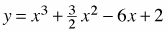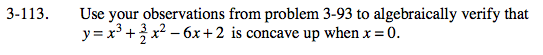### Home > CALC > Chapter 3 > Lesson 3.3.3 > Problem3-113

3-113.

Use your observations from problem 3-98 to algebraically verify thatis concave up when x = 0. Homework Help ✎Your study team probably observed that concavity has to do with the way that slopes change.

If f '(x) increases, then the graph of f(x) is curving up and will be concave up.
If f '(x) decreases, then the graph of f(x) is curving down and will be concave down.

To determine if f(x) is concave up at x = 0, you can test the slope at points before and after x = 0 and see if those slopes are increasing or decreasing.

Find f '(x) and choose points close to x = 0.
Evaluate those points. For example, f '(−0.1) = ___________ and f '(0.1) = __________
Are they increasing or decreasing?

If f '(−0.1) < f '(0.1) then the slopes of f(x) are curving down and the f(x) is concave up at x = 0.
If f '(−0.1) > f '(0.1) then the slopes of f(x) are curving down and the f(x) is concave down at x = 0.